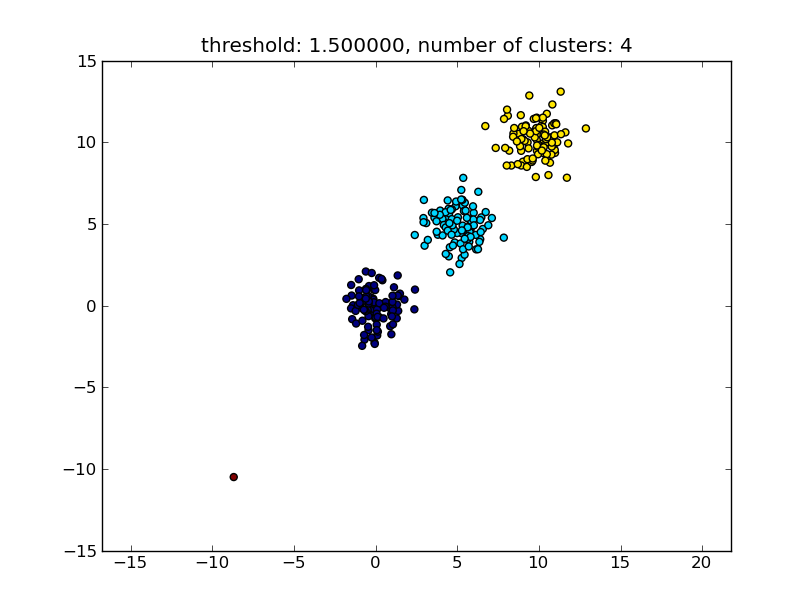1 view

I have a large set of vectors in 3 dimensions. I need to cluster these based on Euclidean distance such that all the vectors in any particular cluster have a Euclidean distance between each other less than a threshold "T".

I do not know how many clusters exist. In the end, there may be individual vectors existing that are not part of any cluster because its Euclidean distance is not less than "T" with any of the vectors in the space.

What existing algorithms/approaches should be used here?

by (33.1k points)

You can simply use Hierarchical Clustering for this problem.

Code:

import matplotlib.pyplot as plt

import numpy

import scipy.cluster.hierarchy as hcluster

# generate 3 clusters of each around 100 points and one orphan point

N=100

data = numpy.random.randn(3*N,2)

data[:N] += 5

data[-N:] += 10

data[-1:] -= 20

# clustering

thresh = 1.5

clusters = hcluster.fclusterdata(data, thresh, criterion="distance")

# plotting

plt.scatter(*numpy.transpose(data), c=clusters)

plt.axis("equal")

title = "threshold: %f, number of clusters: %d" % (thresh, len(set(clusters)))

plt.title(title)

plt.show()

The above for hierarchical clustering will form clusters as shown in this image:There is a threshold given as a parameter, is a distance value on which basis the decision is made so that data points/clusters will be merged into another cluster. The distance metric is used for clustering.

Visit here to know more about Supervised and Unsupervised Machine Learning.